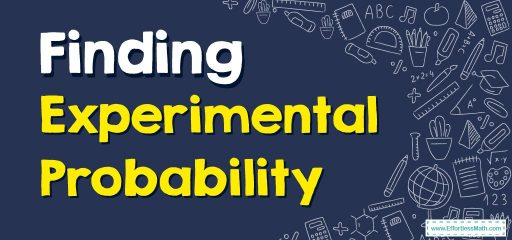# How to Find Experimental Probability?## A step-by-step guide totheexperimental probability

Experimental probability is a probability that is determined based on a series of experiments. A random experiment is conducted and repeated many times to determine its probability, and each repetition is known as a trial. This experiment is done to find the probability of the occurrence or non-occurrence of an event. This could be tossing a coin, rolling a die, or rotating a spinner.

### Experimental probability formula

The experimental probability of an event is based on the number of times the event occurred during the experiment and the total number of times the experiment was performed. Each possible outcome is uncertain and the set of all the possible outcomes is called the sample space. The formula to calculate the experimental probability is:

$$\color{blue}{P\:\left(E\right)=\frac{Number\:of\:times\:an\:event\:occurs}{Total\:number\:of\:times\:the\:experiment\:is\:conducted}}$$

### Experimental probability – Example 1:

The number of cookies made by Sara per day this week is $$4, 8, 6, 10, 5, 10$$, and $$5$$. Based on these data, what is a reasonable estimate of the probability that Sara will make fewer than $$6$$ cookies the next day?

Solution:

$$P(< 6$$ cookies)$$= \frac {3}{7}$$

### What people say about "How to Find Experimental Probability? - Effortless Math: We Help Students Learn to LOVE Mathematics"?

No one replied yet.

X
30% OFF

Limited time only!

Save Over 30%

SAVE $5 It was$16.99 now it is \$11.99